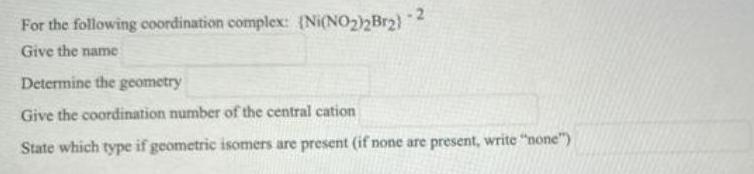Question:

# -2 For the following coordination complex: (Ni(NO₂)2Br2) Give the name Determine the geometry Give the coordination number of the central cation State which type if geometric isomers are present (if none are present, write "none")

Last updated: 8/15/2022For the following coordination complex: (Ni(NO₂)₂Br₂)^-2 Give the name Determine the geometry Give the coordination number of the central cation State which type if geometric isomers are present (if none are present, write "none")